# Common Core: High School - Geometry : Prove Simple Geometric Theorems by using Coordinates: CCSS.Math.Content.HSG-GPE.B.4

## Example Questions

← Previous 1

### Example Question #31 : Expressing Geometric Properties With Equations

True or False:

The pointlies on the ellipse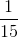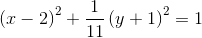True

False

False

Explanation:

In order to solve this problem, we need to plug in the point, and see if both sides of the equation are equal.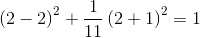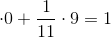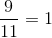The point does not lie on the ellipse, because each side is not equal to each other. So our answer is False.

### Example Question #1 : Prove Simple Geometric Theorems By Using Coordinates: Ccss.Math.Content.Hsg Gpe.B.4

True or False:

The pointlies on the ellipse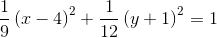True

False

False

Explanation:

In order to solve this problem, we need to plug in the point, and see if both sides of the equation are equal.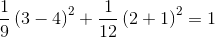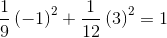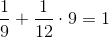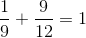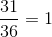The point does not lie on the ellipse, because each side is not equal to each other. So our answer is False.

### Example Question #4 : Prove Simple Geometric Theorems By Using Coordinates: Ccss.Math.Content.Hsg Gpe.B.4

True or False:

The pointlies on a circle with a center ofand a radius.

False

True

False

Explanation:

In order to solve this problem, we need to write out the general equation of a circle.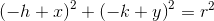Now let's substitute for,, and.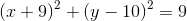Now we can plug in the point, and see if it lies on the circle.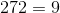The point does not lie on the circle, because each side is not equal to each other. So our answer is False.

### Example Question #1 : Prove Simple Geometric Theorems By Using Coordinates: Ccss.Math.Content.Hsg Gpe.B.4

True or False:

The pointlies on the line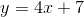True

False

False

Explanation:

In order to solve this problem, we need to plug in the point and see if both sides of the equation are equal or not.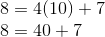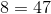The point does not lie in the line, because each side is not equal to each other. So our answer is False.

### Example Question #2 : Prove Simple Geometric Theorems By Using Coordinates: Ccss.Math.Content.Hsg Gpe.B.4

True or False:

The pointlies on the line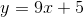True

False

False

Explanation:

In order to solve this problem, we need to plug in the point and see if both sides of the equation are equal or not.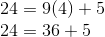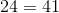The point does not lie in the line, because each side is not equal to each other. So our answer is False.

### Example Question #7 : Prove Simple Geometric Theorems By Using Coordinates: Ccss.Math.Content.Hsg Gpe.B.4

True or False:

The pointlies on the ellipse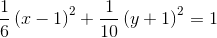True

False

False

Explanation:

In order to solve this problem, we need to plug in the point, and see if both sides of the equation are equal.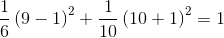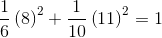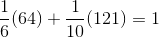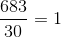The point does not lie on the ellipse, because each side is not equal to each other. So our answer is False.

### Example Question #8 : Prove Simple Geometric Theorems By Using Coordinates: Ccss.Math.Content.Hsg Gpe.B.4

True or False:

The pointlies on a circle with a center ofand a radius.

True

False

False

Explanation:

In order to solve this problem, we need to write out the general equation of a circle.Now let's substitute for,, and.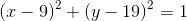Now we can plug in the point, and see if it lies on the circle.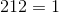The point does not lie on the circle, because each side is not equal to each other. So our answer is False.

### Example Question #9 : Prove Simple Geometric Theorems By Using Coordinates: Ccss.Math.Content.Hsg Gpe.B.4

True or False:

The pointlies on the ellipse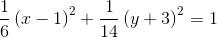True

False

False

Explanation:

In order to solve this problem, we need to plug in the point, and see if both sides of the equation are equal.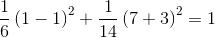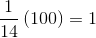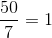The point does not lie on the ellipse, because each side is not equal to each other. So our answer is False.

### Example Question #3 : Prove Simple Geometric Theorems By Using Coordinates: Ccss.Math.Content.Hsg Gpe.B.4

True or False:

The pointlies on the line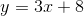True

False

False

Explanation:

In order to solve this problem, we need to plug in the point and see if both sides of the equation are equal or not.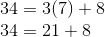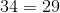The point does not lie in the line, because each side is not equal to each other. So our answer is False.

### Example Question #4 : Prove Simple Geometric Theorems By Using Coordinates: Ccss.Math.Content.Hsg Gpe.B.4

True or False:

The pointlies on the line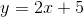True

False

False

Explanation:

In order to solve this problem, we need to plug in the point and see if both sides of the equation are equal or not.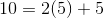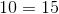The point does not lie in the line, because each side is not equal to each other. So our answer is False.

← Previous 1

### All Common Core: High School - Geometry Resources